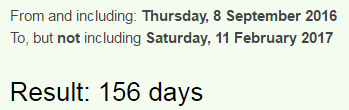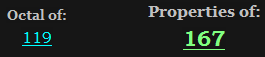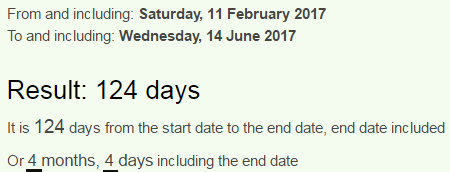CipherFeb 11, 2017

CNN - Bernie Sanders again - Trump is delusional

CNN dropped another funny story today and here we have Sanders criticizing Trump again just 5 days after the last time he appeared (Previous Bernie post)
http://edition.cnn.com/2017/02/10/politics/bernie-sanders-trump-delusional-liar/index.htmlBoth Bernie Sanders and Trump are full of Saturnian coding so it's probably all gonna revolve around 93, 119, 511.....Also Bernie is born in New York which is coded all over with 39 and 93, today he's appearing on "OutFront" = 39Trump is delusional = 2+9+3+4+7 + 9+10 + 4+5+3+3+10+9+6+5+1+3 = 93 (S Exception)
says he could move US into authoritarian mode = 1+1+7+1 +8+5+3+6+3+3+4+4+6+4+5+3+1+9+5+2+6+1+3+2+8+6+9+9+2+1+9+9+1+5+ 4+6+4+5 = 171 (Reduced)Bernie Sanders is showing up 156 days after his birthday, 156th prime number is 911Gematria of his birthday connects to the date of this story, his name and his nick name
September Eighth = 1+5+7+2+5+4+2+5+9 + 5+9+7+8+2+8 = 79 (Reduced)
February Eleventh = 6+5+2+9+3+1+9+7 + 5+3+5+4+5+5+2+8 = 79 (Reduced)
2/11 - can be reduced to 2/2 (22nd prime number is 79)
Bernie Sanders = 2+5+9+5+9+5 + 10+1+5+4+5+9+10 = 79 (S Exception)
Bernard Sanders = 2+5+9+5+1+9+4 + 10+1+5+4+5+9+10 = 79 (S Exception)
May First comes 79 days after February Eleventh, and if you've been following my recent posts you know what I'm referring to, it's a lot to get into right nowThe video is 56 seconds long - President = 56 (S exception)
The woman interviewing him is called Erin Burnett
Erin Burnett = 5+9+9+5 + 2+3+9+5+5+2+2 = 56 (Reduced)
Erin = 5+9+9+5 = 28 (Reduced) , Burnett = 2+3+9+5+5+2+2 = 28 (Reduced)
He is criticizing Trump on a date with 23 and 50 numerology both correlating to the name Donald
2/11/2017 - 2+11+2+0+1+7 = 23 Donald = 4+6+5+1+3+4 = 23 (Reduced)
2/11/2017 = 2+11+20+17 = 50 Donald = 4+15+14+1+12+4 = 50 (Ordinal)Sanders is a frequent Trump critic = 10+1+5+4+5+9+10+9+10+1+6+9+5+8+3+5+5+2+2+9+3+4+7+3+9+9+2+9+3 = 167 (S Exception)
167 is 39th prime number, but it also connects to 119 through octal system, so it's a very interesting numberThe opening line always has something coded into it
Sen. Bernie Sanders says he doesn't like calling President Donald Trump "delusional" -- but believes the President's actions call for it.318 is of the special numbers to watch out for - Bernie = 12+30+108+84+54+30 = 318 (Sumerian)Sanders = 10+1+5+4+5+9+10 = 44 (S Exception)
This comes 4 months and 4 days before Trump's birthdayBernie is gonna be coming up all year to criticize Trump it seems haha
Sanders is a frequent Trump critic = 19+1+14+4+5+18+19 + 9+19 + 1 + 6+18+5+17+21+5+14+20 + 20+18+21+13+16 + 3+18+9+20+9+3 = 365 (Ordinal)